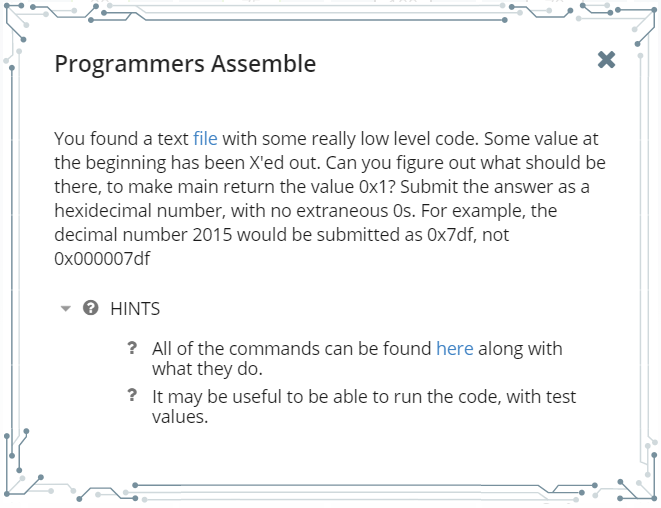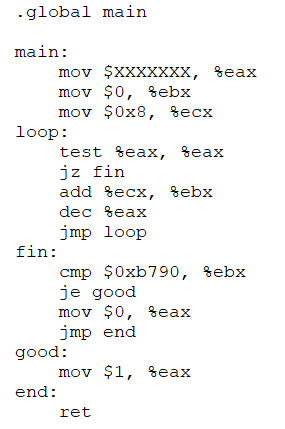# PicoCTF 2017 – Programmers Assemble #reverseengineering #assembly #infosec

Another day, another hacking challenge.

In today’s challenge we’re going to focus on a reverse engineering exercise.

Clicking on the exercise we see the following:Assembly… eeekk!

Let’s break it down – line by line.

eax = ???

ebx = 0

ecx = 8

for the line test %eax, %eax we’re testing to see if the zero flag of the %eax register is equal to zero

When eax is zero then we jump to the fin label, and compare ebx to see if it’s 46992.

So what do we need to set eax so ebx will be 46991? What happens if we divide 46992/8? Could this possibly give us the value of eax? Dividing 46991/8 = 5,874 decimal = 0x16F2.

You may be wondering, why would you divide 46992 by 8?

Looking at the assembly we know that when eax is not zero, we add 8 to the ebx register, and decrement eax by one and jump back to the loop label. We know if we set eax to 0x16F2 or 5,874 decimal then when eax equal 0 the program will jump to the fin label and the ebx register will be 0xb6790 or 46991 decimal. Once the comparison happens the program will jump again to the good label which will assign the eax register to 1.

To put this in a computer language it will be:

eax = 5874;

ebx = 0;

ecx = 8;

if(eax ==0) {

if(ebx == 46991) {

eax = 1;

} else {

eax = 0;

}

else {

ebx = ebx + ecx;

}

eax–;

Moral of the story assembly is the bare bones of ALL programming languages. No matter what language you use it can be broken down into assembly.

Entering 0x16F2 into the text box we acquired 75 points!